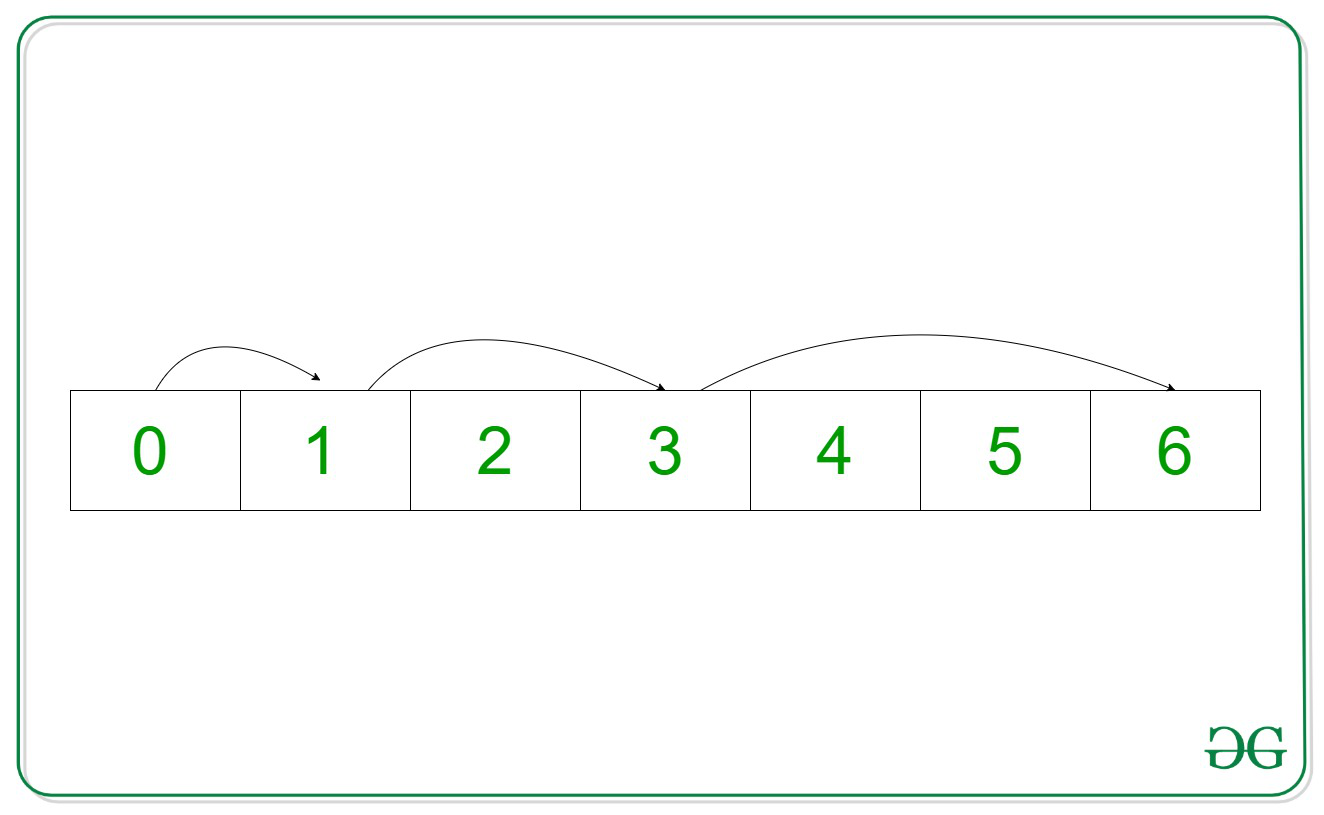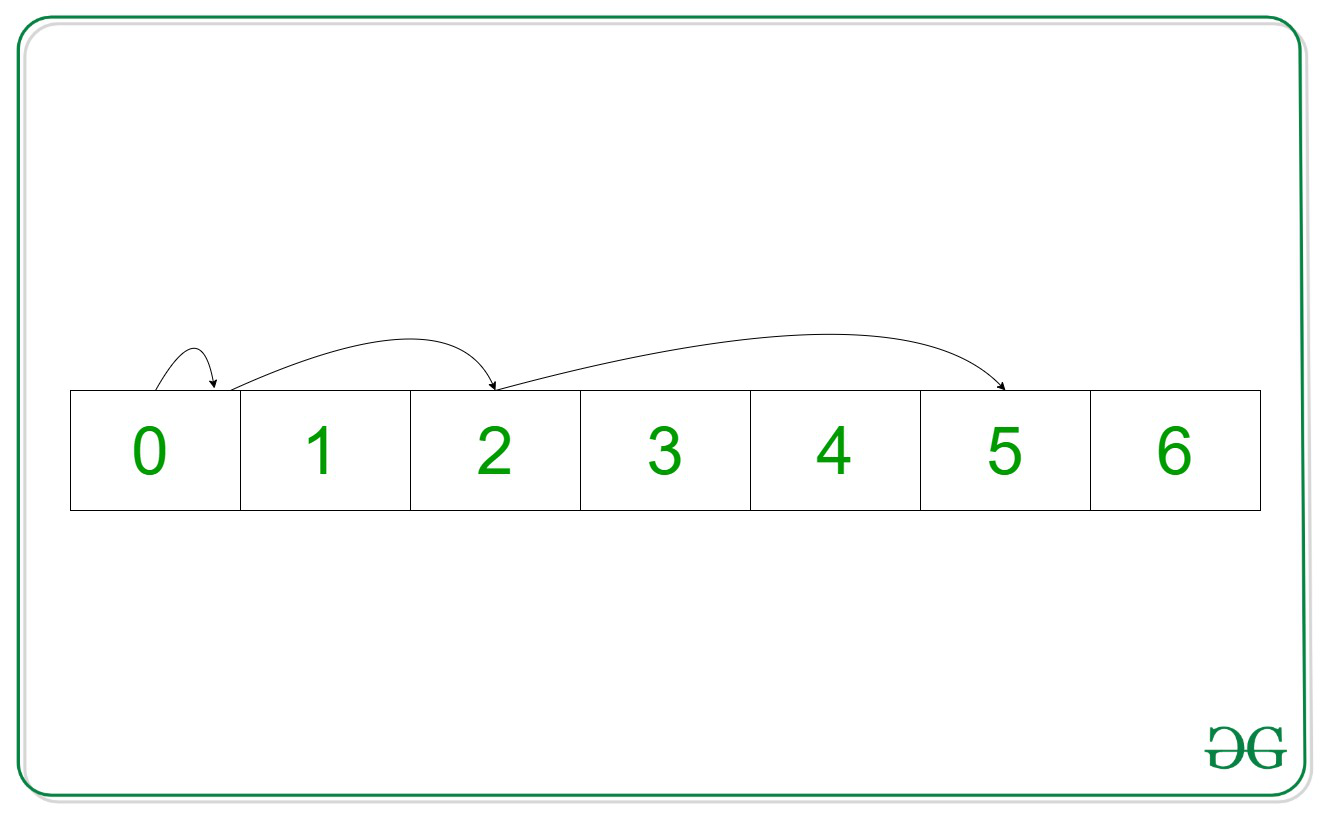# Maximum index a pointer can reach in N steps by avoiding a given index B – Set 3 (Binary Search)

• Difficulty Level : Easy
• Last Updated : 07 Oct, 2021

Given two integers N and B, the task is to print the maximum index a pointer, starting from 0th index can reach in an array of natural numbers(i.e., 0, 1, 2, 3, 4, 5…), say arr[], in N steps without placing itself at index B at any point.

In each step, the pointer can move from the Current Index to a Jumping Index or can remain at the Current Index.
Jumping Index = Current Index + Step Number

Attention reader! Don’t stop learning now. Get hold of all the important DSA concepts with the DSA Self Paced Course at a student-friendly price and become industry ready.  To complete your preparation from learning a language to DS Algo and many more,  please refer Complete Interview Preparation Course.

In case you wish to attend live classes with experts, please refer DSA Live Classes for Working Professionals and Competitive Programming Live for Students.

Examples:

Input: N = 3, B = 2
Output: 6
Explanation:Step 1:
Current Index = 0
Step Number = 1
Jumping Index = 0 + 1 = 1
Step 2:Current Index = 1
Step Number = 2
Jumping Index = 1 + 2 = 3
Step 3:
Current Index = 3
Step Number = 3
Jumping Index = 3 + 3 = 6
Therefore, the maximum index that can be reached is 6.

Input: N = 3, B = 1
Output: 5
Explanation:Step 1:
Current Index = 0
Step Number = 1
Jumping Index = 0 + 1 = 1But this is bad index. So pointer remains at the Current Index.
Step 2:
Current Index = 0
Step Number = 2
Jumping Index = 0 + 2 = 2
Step 3:
Current Index = 2
Step Number = 3
Jumping Index = 2 + 3 = 5
Therefore, the maximum index that can be reached is 5.

Efficient Approach: The naive approach discussed in this article can also be optimized by using Binary Search. If the value of maximumIndex – B exists in the sum of any last K numbers from the first N natural numbers then the maximumIndex can’t be reduced to less than equal to 0 without the jump on index B. therefore, decrement the maximum Index by and perform the above steps again. Follow the steps below to solve the problem:

Below is the implementation of the above approach:

## C++

 `// C++ program for the above approach``#include ``using` `namespace` `std;` `// Function to find the maximum index``// reachable``int` `maxIndex(``int` `N, ``int` `B)``{` `    ``// Store the answer``    ``int` `maximumIndexReached = 0;``    ``vector<``int``> Ans;` `    ``// Store the maximum index possible``    ``// i.e, N*(N-1)``    ``for` `(``int` `i = 1; i <= N; i++) {``        ``maximumIndexReached += i;``        ``Ans.push_back(i);``    ``}` `    ``reverse(Ans.begin(), Ans.end());` `    ``// Add bthe previous elements``    ``for` `(``int` `i = 1; i < (``int``)Ans.size(); i++) {``        ``Ans[i] += Ans[i - 1];``    ``}` `    ``// Run a loop``    ``while` `(maximumIndexReached) {` `        ``// Binary Search``        ``auto` `it``            ``= lower_bound(Ans.begin(), Ans.end(),``                          ``maximumIndexReached - B);``        ``if` `(it == Ans.end()) {``            ``break``;``        ``}``        ``if` `(*it == maximumIndexReached - B) {``            ``maximumIndexReached--;``        ``}``        ``else` `{``            ``break``;``        ``}``    ``}` `    ``return` `maximumIndexReached;``}` `// Driver Code``int` `main()``{``    ``int` `N = 3, B = 2;``    ``cout << maxIndex(N, B);` `    ``return` `0;``}`

## Python3

 `# Python 3 program for the above approach``from` `bisect ``import` `bisect_left` `# Function to find the maximum index``# reachable``def` `maxIndex(N, B):` `    ``# Store the answer``    ``maximumIndexReached ``=` `0``    ``Ans ``=` `[]` `    ``# Store the maximum index possible``    ``# i.e, N*(N-1)``    ``for` `i ``in` `range``(``1``, N ``+` `1``):``        ``maximumIndexReached ``+``=` `i``        ``Ans.append(i)` `    ``Ans.reverse()` `    ``# Add bthe previous elements``    ``for` `i ``in` `range``(``len``(Ans)):``        ``Ans[i] ``+``=` `Ans[i ``-` `1``]` `    ``# Run a loop``    ``while` `(maximumIndexReached):` `        ``# Binary Search``        ``it ``=` `bisect_left(Ans,``                         ``maximumIndexReached ``-` `B)``        ``if` `(it ``not` `in` `Ans):``            ``break` `        ``if` `(it ``=``=` `maximumIndexReached ``-` `B):``            ``maximumIndexReached ``-``=` `1` `        ``else``:``            ``break` `    ``return` `maximumIndexReached` `# Driver Code``if` `__name__ ``=``=` `"__main__"``:` `    ``N ``=` `3``    ``B ``=` `2``    ``print``(maxIndex(N, B))` `    ``# This code is contributed by ukasp.`

## Javascript

 ``

Output:
`6`

Time Complexity: O(N*log N)
Auxiliary Space: O(N)

My Personal Notes arrow_drop_up